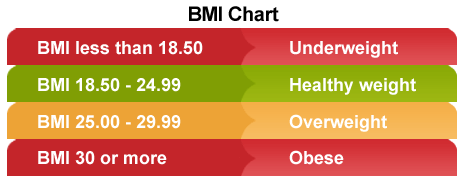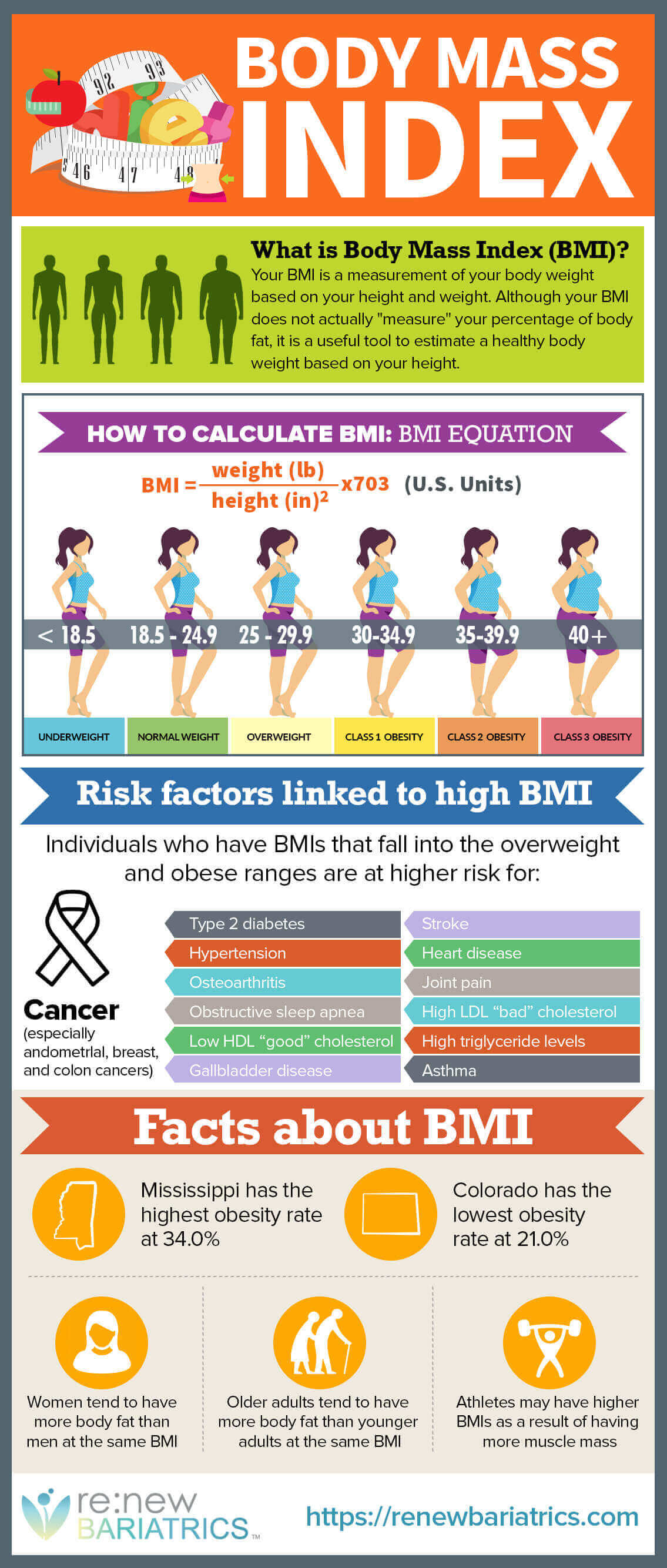So you’ve decided to lose weight and reach the goal weight that you had before, but don’t know where to start? Don’t rush with choosing any weight loss program or heading to the nearest fitness club. If you are seriously concerned about your weight, the first step is to find out the level of obesity. To assess weight there are many methods and formulas. One of the most popular checks to calculate ideal weight is the Quetelet formula that allows calculating body mass index (BMI).Infographic Source: https://renewbariatrics.com/bmi-body-mass-index/

Anyone who searches online for topics such as “how to lose weight” and “how to lose weight fast”, neglect the concept of “body mass index” (BMI). BMI allows you to define obesity, or, in the opposite, lack of weight.

Body mass index is calculated by the following formula BMI = m (kg): N * n (m2), more simply — human weight measured in kilograms, divided by the square of the growth, measured in meters.

To illustrate this, we look at an example of calculation. Let’s assume that someone has height 1 meter 77 centimeters and weigh 66 kilograms.

Mass index formula Quetelet is calculated in two steps:

1.77 * 1.77 = 3132
66: 3132 ~ 21% (approx.)

It turns out that with the growth of 177 cm and weighing in at 66 pounds, BMI (body mass index) is 21.If these calculations are too complicated for you, you can find some existing body mass index calculator, for example this one.

Body mass index is an indicator of your body’s health and can used as an indicator for your risk for developing serious medical conditions.

For men the normal range for BMI is 19 – 25, for women the normal mass index is in the range of 19 -24.

If your BMI is less than 18.5 – your weight is below the normal range and losing additional weight is not recommended.

BMI 25.0-29.9 speaks about excess body weight. This stage is called “overweight”.
BMI 30.0-34.9 obesity – 1 degree, dangerous risk for Comorbidities.

35.0 – 39.9 BMI obesity – 2 degrees. Recommended to proceed immediately to weight correction.

35.0-39.9 BMI is obesity – 3 degrees. There is a very high risk of associated diseases; therefore, reduction of weight, even if not to a perfect value, is an urgent need. And it is better to undergo weight loss under physician supervision.

When calculating the body mass index, you need to take into account the type of physique you have. The figures above are suitable for mesomorphic (people with “normal” width of bone) individuals. For ectomorphic (“narrow bone”) individuals, rule BMI can be reduced to 19 and for endomorphic (“wide bone») increased to 33.

The formula for calculating the Quetelet index weight is suitable for analyzing the weight of females and males aged 20 and up to 65 years. It is not recommended to determine the ideal weight for pregnant women, lactating women, teens or athletes. BMI calculation use absolute value of the body mass, and does not take into account the amount of fat and muscle mass. Therefore, body mass index should be used with caution.